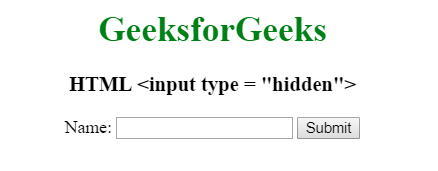# HTML <input type=”hidden”>

• Last Updated : 22 Jul, 2022

The HTML <input type=”hidden”> is used to define a input Hidden field. A hidden field also includes those data that could not be seen or modified by the users when submitted the form. A hidden field only stores those database records that need to be updated when submitting the form.

Syntax:

`<input type="hidden"> `

Example:

## html

 ```<``html``>` `<``head``>``    ``<``title``>``        ``HTML input type hidden``    ````    ` `    ``<``style``>``        ``h1 {``            ``color: green;``        ``}``        ``body {``            ``text-align: center;``        ``}``    ````` `<``body``>``    ``<``h1``>``        ``GeeksforGeeks``    ````    ` `    ``<``h3``>``        ``HTML <input type = "hidden">``    ````    ` `    ``<``form` `action="#">``        ``<``input` `type="hidden" id="myFile" value="1234">``    ` `        ``Name: <``input` `type="text">``        ` `        ``<``input` `type="submit" value="Submit">``    ````` `                                   `

Output:Supported Browsers: The browsers supported by <input type=”hidden”> are listed below:

• Google Chrome 1.0 and above
• Edge 12.0 and above
• Internet Explorer
• Firefox 1.0 and above
• Safari 1.0 and above
• Opera 2.0 and above

My Personal Notes arrow_drop_up